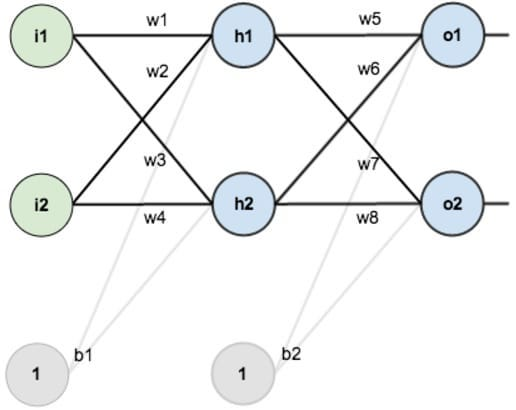# Understanding Neural Networks from Scratch

Original article can be found here (source): Deep Learning on Medium# Understanding Neural Networks from Scratch

Have you ever wondered how does our brain recognizes and remembers everything around us.This is because particular neurons in our brain fire at same time whenever we see,hear,taste,smell,touch something.Our mind is trained for such things during our lifetime.Our brain contains billions of neurons where each cluster of neurons acts as a biological neural network.Our scientists inspired by this unique ability of our brain and designed an Artificial Neural Network.

# Artificial Neural Network

An Artificial Neural Network is Mathematical Model which contains a group of Artificial neurons connected to each other.In simple words ANN is a non-linear modelling of statistical data.An ANN consist of three parts :

1. Input Layer
2. Hidden Layer
3. Output Layer

Each layer consists certain no of neurons and each neuron of a layer is connected to each neuron of the next layer.

Each connection between two neurons is associated with a weight which multiplies with the previous layer activation.Each hidden layer neuron is activated by a non-linear activation function and a bias.

# Training a Neural Network

During our childhood we learn to recognize animals,fruits,objects etc by seeing their pictures for multiple times.We recognize patterns in each picture to remember them.While we are learning alphabets in our childhood we make several mistakes writing them.In each mistake our brain is getting trained to write the alphabet correctly next time.The training of a neural network is quiet similar to how we train our brain.

So we need a training data in order to perform a particular task using a neural network.

# Math behind Neural Networks

In order train a neural network we need a lot of math.So lets dive into all the mathematical terminologies used for training a neural network.

1. Activation Function
2. Error Function
3. Cost Function
4. Forward propagation

## Activation Functions

The main aim of a neural network is to learn patterns in a given data.Patterns are non linear functions so we need non-linear activation functions to learn non linear patterns.Different Activation functions are used depending on the task.

## Error Functions

As we learnt neural network learns from mistakes.So we need a metric to measure mistakes done by neural networks.We calculate the mistakes in the form of error. Error function is the function of ground truths and output of neural network.Different error functions are used depending on the task.Mean square error is generally used for regression,Cross entropy loss is used for classification task.

## Cost Functions

A Cost Function is used for evaluating the performance of a neural network.Different cost functions are used depending the task.Some of the cost functions are mentioned below

## Forward Propagation

Lets dive into the math on how information is transmitted through each neuron in the neural network from input layer to output layer.

Lets generalize forward propagation for a N-layered Neural Network.

## Back-propagation

As we said neural networks learn from their mistakes .So to reduce the mistakes done we need to optimize the cost function of neural network.Let J be the cost function .Cost Function is a function of weights and bias of the network.So we need to find the local minima of the cost function w.r.t weights and bias of the network.